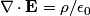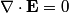## Solution to 1986 Problem 80

 The electric field must obey Gauss's Law\begin{align*}\nabla \cdot \mathbf{E} = \rho/\epsilon_0\end{align*}or\begin{align*}\nabla \cdot \mathbf{E} = 0\end{align*}since there are no charges in the region. Only answer (B) is divergenceless, hence answer (B) is the correct one.